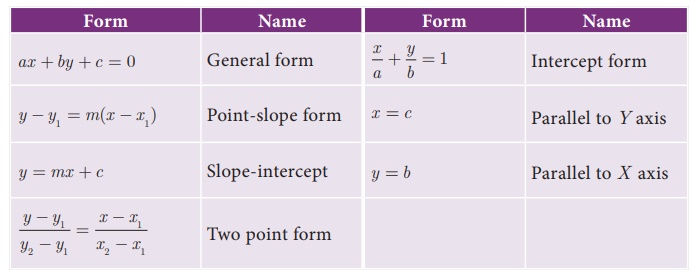Home | | Maths 10th Std | Points to Remember

# Points to Remember

Mathematics : Coordinate Geometry: Points to Remember: Equation, Formulas, forms

Points to Remember

·           The area of a triangle formed by the points (x1 , y1 ) , (x2 , y2 ) and (x3 , y3 ) is 1/2 { (x1y2 + x2y3 + x3 y1) (x2y1 + x3y2 + x1y3) } sq.units

·           Three points A(x1,y1),B(x2,y2) and C(x3,y3) are collinear if and only if

(i) area of ΔABC = 0 or x1y2 + x2y3 + x3y1 = x2y1 + x3y2 + x1y3

(ii) slope of AB = slope of BC or slope of AC

·           The area of a quadrilateral formed by the four points  (x1 , y1 ) , (x2 , y2 ), (x3 , y3 ) and (x4 , y4 )is 1/2 { (x1y2 + x2y3 + x3 y4+ x4 y1) (x2y1 + x3y2 + x4y3+ x1y4) } sq.units

·           If a line makes an angle θ with the positive direction of X axis, then its slope m = tanθ.

·           If A(x1,y1), B(x2,y2) are two distinct points then the slope of AB is (y2 - y1) / (x2 - x1).

·           Slope of line ax+by+c= 0 is m =  − a/b.

Equation of straight line in various forms·           Two straight lines are parallel if and only if their slopes are equal.

Two straight lines with well defined slopes m1, m2 are perpendicular if and only if m1×m2 = −1.

Tags : Coordinate Geometry | Mathematics , 10th Mathematics : UNIT 5 : Coordinate Geometry
Study Material, Lecturing Notes, Assignment, Reference, Wiki description explanation, brief detail
10th Mathematics : UNIT 5 : Coordinate Geometry : Points to Remember | Coordinate Geometry | Mathematics